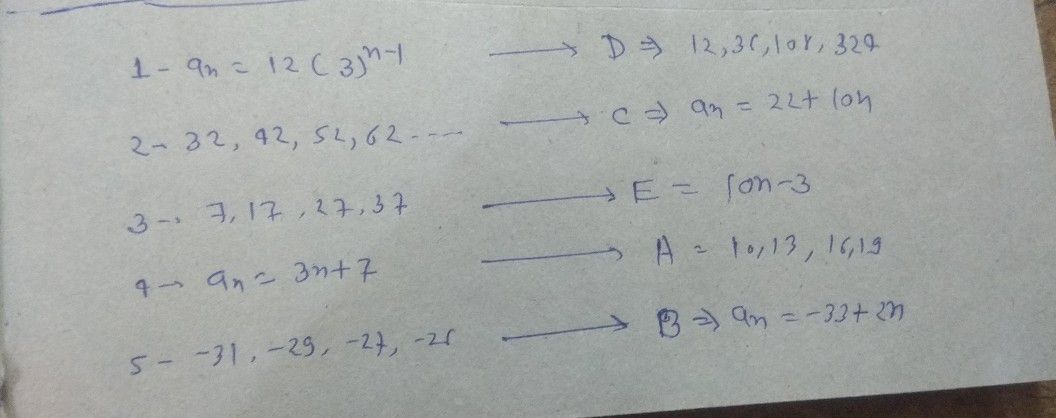Symbol
ProblemAssessment 2: $Direcllons:$ Match column A with the correct answer on column B. Write the letter of th correct answer on the space provided before each number Column A Column B $1.a_{n}=12\left(3\right)^{n-1}$ A. $10,$ $13$ $16,$ $19$ $2.32$ $42$ $0$ $52,$ $62,$ ... B. $a_{n}=-33+2n$ $3.7,$ $17.27$ $37,.$ C. $a_{n}=22+10n$ 4. $a_{n}=3n+7$ D. $12,36,108,324,...$ 5. $-31,-29,-27,-25,$ E. $a_{n}=10n-3$
10th-13th grade
Algebra
Search count: 105
SolutionQanda teacher - yyyygk97Student
Thank you ❤️Qanda teacher - yyyygk97
??